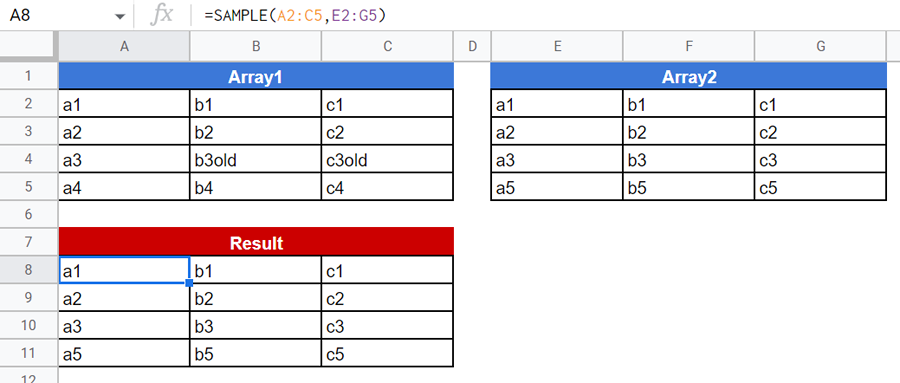# Updating Array1 with Array2 using Google Apps Script

GistsThis is a sample script for updating Array1 with Array2 using Google Apps Script.

As a sample situation, there are 2 arrays (Array1 and Array2) of the 2-dimensional array. The sample situation can be seen in the above sample Spreadsheet.

• Conditions
• When the values of column “A” of Array2 are existing in column “A” of Array1, the rows of Array1 are updated by that of Array2.
• When the values of column “A” of Array2 are not existing in column “A” of Array1, the rows of Array2 are appended to Array1.
• When the values of column “A” of Array1 are not existing in column “A” of Array2, the rows of Array1 are deleted.

I sometimes see such questions on Stackoverflow. So, I thought that when this sample script is posted, it might be useful for users.

## Sample script

function SAMPLE(array1, array2) {
const [obj1, obj2] = [array1, array2].map((e) =>
e.reduce((o, r) => ((o[r] = r), o), {})
);
return [
...array1.reduce((ar, r) => (obj2[r] && ar.push(obj2[r]), ar), []),
...array2.reduce((ar, r) => (obj1[r] || ar.push(r), ar), []),
];
}


When this script is used for the above sample script by putting a custom function =SAMPLE(A2:C5,E2:G5) into a cell, the above situation can be obtained.

## Testing

You can also test this script at https://jsfiddle.net/680h5x9c/.

When Array1 and Array2 are as follows,

const array1 = [
["a1", "b1", "c1"],
["a2", "b2", "c2"],
["a3", "b3old", "c3old"],
["a4", "b4", "c4"],
];
const array2 = [
["a1", "b1", "c1"],
["a2", "b2", "c2"],
["a3", "b3", "c3"],
["a5", "b5", "c5"],
];


The following result is obtained.

[
["a1", "b1", "c1"],
["a2", "b2", "c2"],
["a3", "b3", "c3"],
["a5", "b5", "c5"]
]


## Note

• This sample script can be also used with Javascript and Node.js.